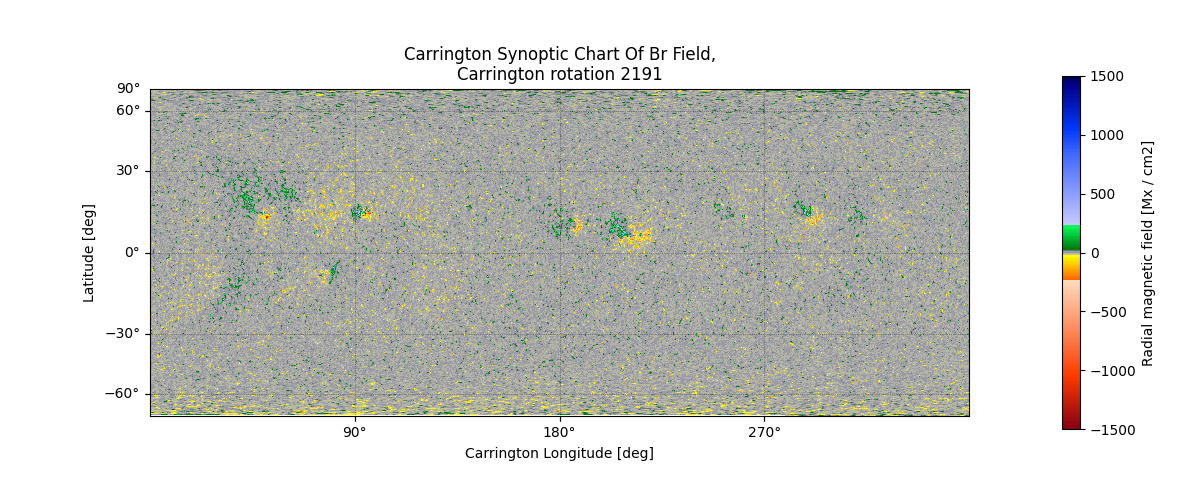In this example we load the Daily Synoptic Maps produced by the HMI team. This data is an interesting demonstration of SunPy’s Map class as it is not in the more common Helioprojective coordinate system, it is in Heliographic Carrington coordinates and in a non-trivial Cylindrical Equal Area projection.

```import numpy as np
import matplotlib.pyplot as plt

import sunpy.map
```

```filename = download_file(
'http://jsoc.stanford.edu/data/hmi/synoptic/hmi.Synoptic_Mr.2191.fits', cache=True)
syn_map = sunpy.map.Map(filename)
```

There are a couple of oddities with this file, firstly the value of ‘CUNIT2’:

```print(syn_map.meta['CUNIT2'])
```

Out:

```Sine Latitude
```

That is not a unit! What this is telling us is that the latitude coordinate is actually the sine of latitude. According to the Thompson (2006) paper, CUNIT2 should be in degrees and CDELT2 should be multiplied by 180/pi. Also the value of CDELT1 has the wrong sign so let’s fix this.

```syn_map.meta['CUNIT2'] = 'degree'
syn_map.meta['CDELT2'] = 180 / np.pi * syn_map.meta['CDELT2']
syn_map.meta['CDELT1'] *= -1
```

Let’s also fix the plot settings

```syn_map.plot_settings['cmap'] = 'hmimag'
syn_map.plot_settings['norm'] = plt.Normalize(-1500, 1500)
```

Let’s plot the results

```fig = plt.figure(figsize=(12, 5))
axes = plt.subplot(projection=syn_map)
im = syn_map.plot()

# Set up the Sine Latitude Grid
x = axes.coords
y = axes.coords

x.set_coord_type('longitude', coord_wrap=360.)
y.set_coord_type('latitude')

x.set_major_formatter('dd')
y.set_major_formatter('dd')

x.set_axislabel("Carrington Longitude [deg]")
y.set_axislabel("Latitude [deg]")

x.set_ticks(color='black', exclude_overlapping=True)
y.set_ticks(color='black', exclude_overlapping=True)

axes.coords.grid(color='black', alpha=0.6, linestyle='dotted', linewidth=0.5)